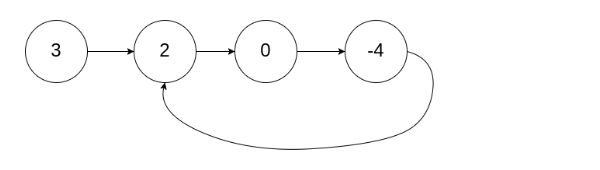Slow and Fast Pointers
0% completed
Slow and Fast Pointer Notes
Delete Middle Node
Add First and Second Reversed Half
Delete Kth node from end in the list
Detect and Remove Cycle7

# Detect And Remove Cycle

Difficulty: EASYContributed By
Sakshi Bansal
Avg. time to solve
10 min
Success Rate
90%

Problem Statement
Suggest Edit

#### A cycle occurs when a node's ‘next’ points back to a previous node in the list.

##### Input Format :
``````The first line of input contains a single integer T, representing the number of test cases or queries to be run.

The first line of each test case contains the elements of the singly linked list separated by a single space and terminated by -1 and hence -1 would never be a list element.

The second line contains the integer position "pos" which represents the position (0-indexed) in the linked list where the tail connects to. If "pos" is -1, then there is no cycle in the linked list.
``````
##### Output Format :
``````For each test case, print two lines.

The first line contains 'True' if the linked list has a cycle, otherwise 'False'.

The second line contains the elements of the singly linked list separated by a single space and terminated by -1. Hence, -1 would never be a list element.
``````
##### Note :
``````You don't have to explicitly print anything yourself. It has been taken care of. Just implement the given function.
``````
##### Constraints :
``````1 <= T <= 10
1 <= N <= 5 * 10^4
-1 <= pos < N
-10^9 <= data <= 10^9 and data != -1

Where 'N' is the size of the singly linked list, and "data" is the Integer data of the singly linked list.
``````
##### Sample Input 1 :
``````2
3 2 0 -4 -1
1
1 2 -1
-1
``````
##### Sample Output 1 :
``````True
3 2 0 -4 -1
False
1 2 -1
``````
##### Explanation for Sample Input 1 :
``````In the 1st test case, there is a cycle, from index 1 -> 2 -> 3 -> 1. The cycle's length is 3.
````````````In the 2nd test case, there is no cycle.
``````
##### Sample Input 2 :
``````2
1 1 1 -1
0
3 -3 -1
-1
``````
##### Sample Output 2 :
``````True
1 1 1 -1
False
3 -3 -1
``````Console